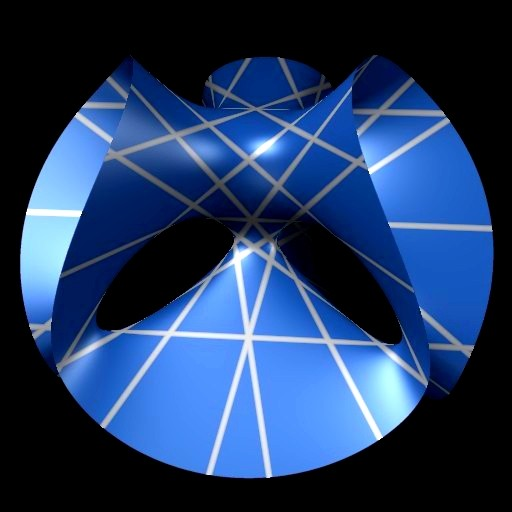# Clebsch's cubic

Here is a smooth surface of degree three, Clebsch's cubic. There are exactly 27 lines on the surface (as on every smooth cubic surface in projective three-space).In projective three-space with homogeneous coordinates (x:y:z:w), Clebsch's cubic is given by the equation

x3 + y3 + z3 + w3 - (x+y+z+w)3 = 0

The nice computer picture shown above was made by Stephan Endrass using his program SURF. (SURF can draw pictures of a surface in three-space when you provide an equation of the surface.)

If you are interested in a more »modern« surface, don't forget to have a look at Barth's sextic.